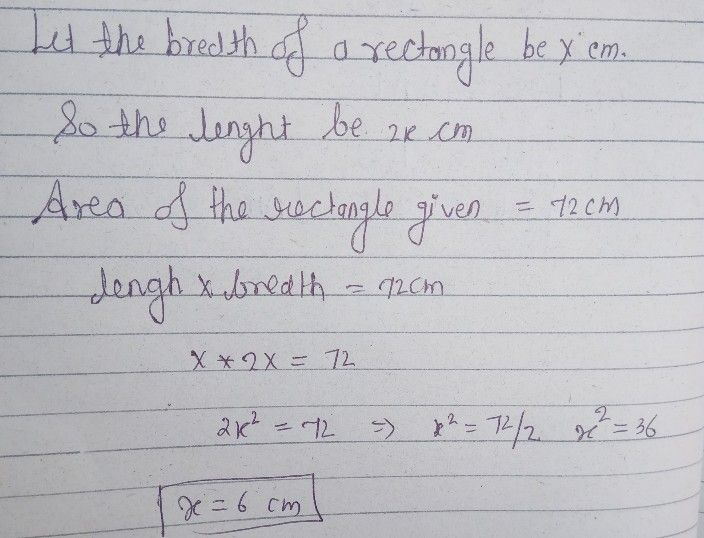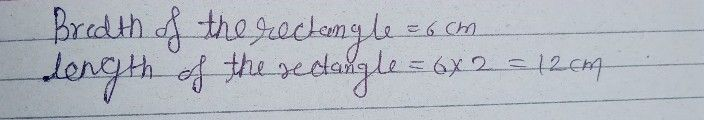Symbol
ProblemSolve the following problems. Show your complete solutions. $7\right)$ The length of a rectangle is twice its width. Its area $is72cm^{2}$ Find the length and width of the rectangle. $\right)$ he perimeter of a rectangle is $34mm$ and its area $is72mm^{2}$ Find its dimensions. he width of a rectangle is $6cm$ shorter than its length. Its area $is40cm^{2}$ Find the length and width of the rectangle.
Other
Search count: 118
SolutionQanda teacher - RASHMIKAany doubt so pls ask me to know
kindly evaluate ans
thanks
we are only one question allow at time by qanda police
if you have more questions so pls ask on preferred match on my profileStudent
thank you ?